## ML Aggarwal Class 6 Solutions for ICSE Maths Chapter 14 Mensuration Ex 14.2

Question 1.
Find the area of the region enclosed by the following figures by counting squares:Solution:(iii) Fill-filled squares = 10
∴ Total area = Area covered by full squares
= 10 × 1 sq. unit = 10 sq. units

(iv) Full-filled squares = 4
Half-filled squares = 4
Area covered by full squares = 4 × 1 sq. unit = 4 sq. units
Area covered by half squares = 4 × $$\frac{1}{2}$$ sq. units = 2 sq. units
∴ Total area = 4 sq. units + 2 sq. units = 6 sq. units

(v) Full-fulled squares = 2 Half-filled squares = 4
Area covered by full squarees = 2 × 1 sq. unit = 2 sq. units
Area covered by half squares = 4 × $$\frac{1}{2}$$ sq. unit = 2 sq. units
∴ Total area = 2 sq. units + 2 sq. units = 4 sq. units.

(vi) Full-filled squares = 3
Half-filled squares = 6
Area covered by full squares = 3 × 1 sq. unit = 3 sq. units
Area covered by half squares = 6 × $$\frac{1}{2}$$ sq. unit = 3 sq. units
∴ Total area = 3 sq. units + 3 sq. units = 6 sq. units

Question 2.
Find the area of the following closed figures by counting squares:Solution: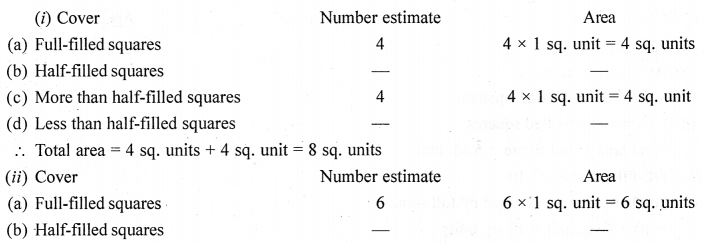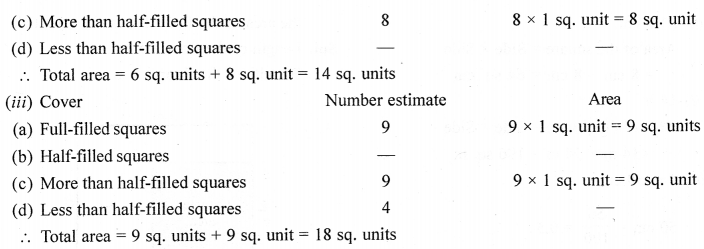Question 3.
Find the areas of the rectangles whose lengths and breadths are:
(i) 9 m and 6 m
(ii) 17 m and 3 m
(iii) 14 m and 4 m
Which one has the largest area and which one has the smallest area?
Solution:
(i) Area of the rectangle = Length × Breadth = 9 m × 6 m = 54 sq. m
(ii) Area of the rectangle = Length × Breadth = 17 m × 3 m = 51 sq. m
(iii) Area of the rectangle = Length × Breadth = 14 m × 4 m = 56 sq. m
The rectangle (iii) has the lartgest area and rectangle (ii) smallest area.

Question 4.
Find the areas of the rectangles whose two adjacent sides are:
(i) 14 cm and 23 cm
(ii) 3 km and 4 km
(iii) 2 m and 90 cm
Solution:
(i) 14 cm and 23 cm
Area of the rectangle = Length × Breadth
= 14 cm × 23 cm
= 322 sq. cm

(ii) 3 km and 4 km
Area of the rectangle = Length × Breadth
= 3 km × 4 km
= 12 sq. km

(iii) 2 m and 90 cm
90 cm = 0.9 m
Area of the rectangle = l × b
= 2 m × 0.9 m
= 1.8 sq. m

Question 5.
Find the areas of the squares whose sides are:
(i) 8 cm
(ii) 14 m
(iii) 2 m 50 cm
Solution:
(i) 8 cm
Area of the square = Side × Side = 8 cm × 8 cm = 64 sq. cm
(ii) 14 m
Area of the square = Side × Side = 14 m × 14 m = 196 sq. m
(iii) 2 m 50 cm
$$50 \mathrm{cm}=\frac{50}{100}=0.5$$ m
∴ Side = 2.5 m
Area of the square = Side × Side = 2.5 m × 2.5 m = 6.25 sq. m

Question 6.
A room is 4 m long and 3 m 25 cm wide. How many square metres of carpet is needed to cover the floor of the room?
Solution:
Length of the room = 4 m
Breadth of the room = 3 m 25 cm = 3.25 m
∴ Area of the room = Length × Breadth = 4 × 3.25 sq. m = 13 sq. m
Hence, 13 square metres of carpet is needed to cover the floor of the room.

Question 7.
What is the cost of tiling a rectangular field 500 m long and 200 m wide at the rate of ₹7.5 per hundred square metres?
Solution:
Length of the rectangular field = 500 m
Breadth of the rectangular field = 200 m
∴ Area of the rectangular field = Length × Breadth
= 500 m × 200 m  = 10000 sq. m
∵ Cost of tiling 100 sq. m = ₹7.5
∴ Cost of tiling 1 sq. m = ₹$$\frac{7.5}{100}$$
∴ Cost of tiling 100000 sq. m = ₹$$\frac{7.5}{100} \times 100000$$ = ₹7500

Question 8.
A floor is 5 m long and 4 m wide. A square carpet of sides 3 m is laid on the floor. Find the area of the floor that is not carpeted.
Solution:
Length of the floor = 5 m
Breadth of the floor = 4 m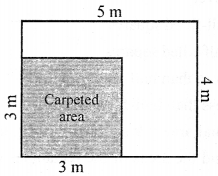∴Area of the floor = Length × Breadth = 5 m × 4 m  = 20 sq m
Area of the square carpet = Side × Side = 3 m × 3 m  = 9 sq m
∴ Area of the floor that is not carpeted = 20 sq m – 9 sq m = 11 sqm

Question 9.
In the given figure, find the area of the path (shown shaded) which is 2 m wide all around.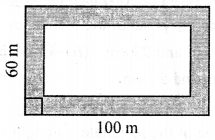Solution:
Length of field = 100 m
Breadth of field = 60 m
Area of the field = L × B = 100 × 60 = 6000m2
Length of field exclude path = 100 – (2 + 2) = 96 m
Breadth of field exclude path = 60 – (2 + 2) = 56
Area of field exclude path = L × B = 96 m × 56 m = 5376 m2
Area of path = Area of field – Area of field exclude path = 6000 – 5376 = 624 sq. m

Question 10.
Four square flower beds of side 1 m 50 cm are dug on a rectangular piece of land 8 m long and 6 m 50 cm wide. What is the area of the remaining part of the land?
Solution:
Area of 1 square flower bed = a= 1.5 × 1.5 = 2.25 m2
Area of 4 square flower bed = 4 × 2.25 = 9 m2
Length of land = 8m
Breadth of land = 6.5 m
Area of land = l × b = 8 × 6.5 = 52 m2
Area of remaining part of bed = 52 – 9 = 43 sq. m

Question 11.
How many tiles whose length and breadth are 12 cm and 5 cm respectively will be needed to cover a rectangular region whose length and breadth are respectively :
(i) 70 cm and 36 cm
(ii) 144 cm and 1 m.
Solution:
(i) Length (l) of tile = 12 cm
breadth (b) of tile = 5 cm
Area of tile = 1 × b = 12 cm × 5 cm = 60 cm2
Length (l) of rectangular region = 70 cm
breadth (b) of rectangular region = 36 cm
Area of rectangular region = l × b = 70 cm × 36 cm = 2520 cm2
If 60 cm2 area is covered then tile required = 1
If 2520 cm2 area is covered then tile required is = $$\frac{1}{60 \mathrm{cm}} \times 2520 \mathrm{cm}^{2}=42$$
Hence, 42 tiles are required.
(ii) Area of the tile = 60 cm2 (as in (i) above)
Length (l) of rectangular region = 1 m = 100 cm
breadth (b) of rectangular region = 144 cm
Area of rectangular region = 100 cm × 144 cm = 14400 cm2
If 60 cm2 are is covered then tile required = 1
If 14400 cm2 is covered then tile required = $$\frac{1}{60 \mathrm{cm}} \times 14400 \mathrm{cm}^{2}=240$$
Hence, 240 tiles are required.

Question 12.
The area of a rectangular plot is 340 sq. m. If its breadth is 17 m, find its length and the perimeter.
Solution:
Area of plot A = 340m2
Length (l) = ?
Area = l × b
⇒ 340 = l × 17
⇒ $$\frac{340}{17}$$
⇒ l = 20
Length = 20 m
Perimeter = 2 (l + b) = 2 (20 + 17) = 2 (37) = 74 m

Question 13.
If the area of a rectangular plot is 144 sq. m and its length is 16 m. Find the breadth of the plot and the cost of fencing it at the rate of ?6 per metre.
Solution:
Area of plot = 144 m2
Length (l) = 16 m.
Area = l × b
⇒ 144 = 16 × b
⇒ $$\frac{144}{16}$$ = b
⇒ b = 9 m
Cost of fencing is ₹6 per metre
Perimeter of field = 2(l + b) = 2 (16 + 9) = 50 m
Cost of fencing = 50 × 6 = ₹300

Question 14.
Split the following shapes into rectangles and find their areas. (The measures are given in centimetres).Solution:
(a) The given figure is split into 2 rectangles.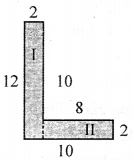Length of Part I = 12 cm
Breadth of Part I = 2 cm
Area of Part I = Length × Breadth = (12 × 2) cm2 = 24 cm2
Length of Part II = 8 cm
Breadth of Part II = 2 cm
Area of Part II = l × b = (8 × 2) cm2 = 16 cm2
∴ Total area = Area of Part I + Area of Part II = (24 + 16) cm2 = 40 cm2

(b) The given figure is divided into 5 partsHere length of all the rectangles = 7 cm
and breadth of all the rectangles = 7 cm
Area = l × b = 7 × 7 = 49 cm2
Total rectangles = 5
∴ Total area = 5 × 49 cm2 = 245 cm2

(c) The given figure is divided into 2 rectangles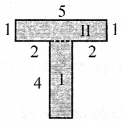Length of Ist Part = 4 cm
Breadth of IInd Part = 1 cm
Area = l × b = 4 × 1 = 4 cm2
Length of IInd Part = 5 cm
Breadth of IInd Part = 1 cm
Area = l × b = 5 × 1 = 5 cm2
∴ Total area = 4 cm2 + 5 cm2 = 9 cm2